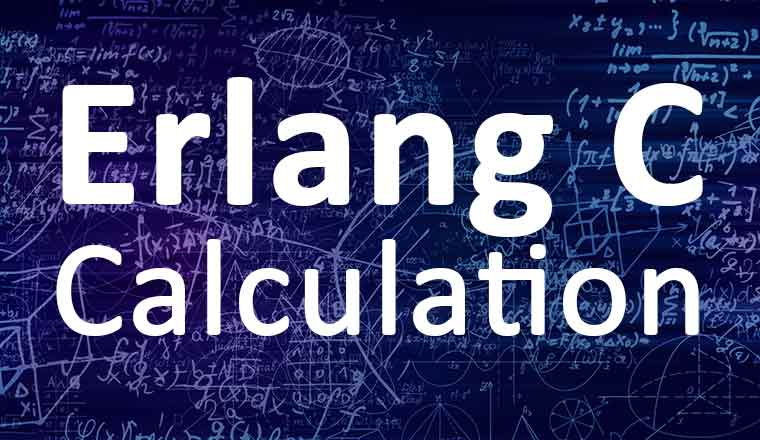# What Is the Erlang C Formula?﻿
1,900

The Erlang C formula is a mathematical equation for calculating the number of agents (advisors) that you need in a call centre, given the number of calls and the service level that you want to achieve.

## Erlang Formula Inputs

• The inputs to the Erlang C formula are
• Incoming Contacts (calls)
• Reporting Period (minutes
• Average Handling Time (AHT) (seconds)
• Required Service Level Percentage

For example you have 400 calls per half hour.  The average time taken to handle a call is 600 seconds and you want to answer 80% of the calls in 20 seconds.

There are also a number of ways to extend the basic formula

• Shrinkage – A factor to include holidays, meetings, training etc
• Maximum Occupancy (usually set to 85%
• Average time to abandon (used in the Erlang A formula)

## Erlang Formula Outputs

The main output of the Erlang C formula is the number of agents needed to handle the contact volume.

## Erlang C Formula Step By Step

The mathematics behind it can be quite complex but there is a good article that describes the Erlang C formula step-by-step.

## The Erlang Calculator

Luckily the easiest way to use the Erlang C formula is to use an erlang calculator.

There are two main types of Erlang Calculator

Author: Robyn Coppell

Published On: 12th May 2022